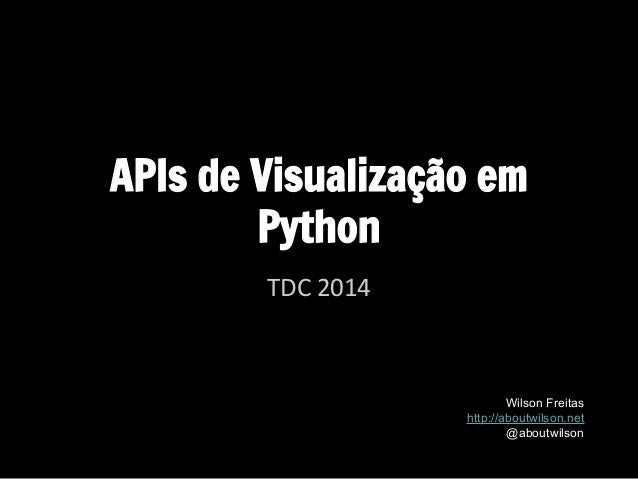Successfully reported this slideshow.Upcoming SlideShare
×

# APIs de Visualização em Python

8,165 views

Published on

Visualização é fundamental para a comunicação em data science. Esta apresentação visa mostrar algumas ferramentas de visualização em Python.

Material apresentado na Trilha Python da TDC 2014 SP (09/Ago/2014).

Published in: Data & Analytics
• Full Name
Comment goes here.

Are you sure you want to Yes No• Be the first to comment

### APIs de Visualização em Python

1. 1. APIs de Visualização em Python TDC 2014 Wilson Freitas http://aboutwilson.net @aboutwilson
2. 2. Visualização
3. 3. Visualização + Python seaborn bokeh ggplot prettyplotlib Vincent Vega (Trifacta) JavascriptSupport Pandas Support
4. 4. ggplot
5. 5. seaborn ● Biblioteca de visualização baseada no matplotlib " seaborn is a library for making attractive and informative statistical graphics in Python " ● Boa para análise exploratória de dados ● Fortemente integrada com pandas e numpy
6. 6. " Bringing Matplotlib to the Browser " ● Integra matplotlib com D3js ● Cria visualização de dados interativa no navegador mpld3
7. 7. Vincent " A Python to Vega translator " " Vega is a visualization grammar, a declarative format for creating, saving and sharing visualization designs. " ● Estruturas de dados Python ● Visualização em JavaScript ● Integração com pandas
8. 8. Bokeh ● Biblioteca de visualização interativa ● Integração com pandas ● Foco: explorar a visualização dos navegadores
9. 9. dados: Top 100 Wines of the World 2014
10. 10. DataFrame pandas: wty
11. 11. Quantos vinhos por País? ggplot(wty, aes("Country")) + geom_bar(fill="red", colour="black") + theme(axis_text_x=element_text(angle=90))
12. 12. Quantos vinhos por País? (com estilo) ggplot(wty, aes("Country")) + geom_bar(fill="red", colour="black") + theme_xkcd() + theme(axis_text_x=element_text(angle=90))
13. 13. Qual a relação entre Pontos e Prêmios? ggplot(wty, aes(x='Prizes', y='Points')) + geom_point()
14. 14. Qual a relação entre Pontos e Prêmios? ggplot(wty, aes(y="Points", x="Prizes")) + geom_point(size=60, shape=6) + stat_smooth(method='lm', colour='red')
15. 15. Qual a relação entre Pontos e Prêmios por País? idx = (wty.Country == 'AUSTRALIA') | (wty.Country == 'FRANCE') | (wty.Country == 'SPAIN') | (wty.Country == 'USA') | (wty.Country == 'ARGENTINA') gplot(wty[idx], aes(x="Prizes", y="Points")) + geom_point() + stat_smooth(method='lm', color='blue') + facet_wrap("Country", scales="fixed")
16. 16. Como se distribuem os prêmios por País? wty_country_prizes = wty['Prizes'].groupby(wty['Country']) idx = wty_country_prizes.count() == 1 wty_t = wty.ix[[c not in idx[idx].index for c in wty.Country], ['Country','Prizes']] wty_t_country_mean = wty_t.groupby(wty_t.Country).mean() country_names = wty_t_country_mean.Prizes.sort(inplace=False).index import seaborn as sb sb.set(style="ticks") f, ax = plt.subplots() sb.offset_spines() sb.violinplot(wty_t['Prizes'], wty_t['Country'], order=country_names) locs, labels = plt.xticks() plt.setp(labels, rotation=45) sb.despine(trim=True)
17. 17. wty_country = wty.groupby('Country') country_count = wty_country['Wine'].aggregate(len) country_count.sort(inplace=True) fig, ax = plt.subplots(figsize=(12,8)) ax.spines['top'].set_visible(False) ax.spines['right'].set_visible(False) ax.spines['left'].set_visible(False) ax.get_xaxis().tick_bottom() ax.get_yaxis().tick_left() plt.subplot(221) g = wty_country['Prizes'].count() x = np.arange(len(g)) countries = list(g.index.values) y = g.values plt.bar(x+1, y, align='center', width=0.5, alpha=0.4) plt.xticks(x+1, [' ']*10, rotation=90) plt.title('Contagem') plt.ylabel('Prizes') plt.subplot(222) g = wty_country['Prizes'].mean() y = g.values plt.bar(x+1, y, align='center', color='red', width=0.5, alpha=0.4) plt.xticks(x+1, [' ']*10, rotation=90); plt.title(u'Média') plt.subplot(223) g = wty_country['Prizes'].max() y = g.values plt.bar(x+1, y, align='center', color='darkgreen', width=0.5, alpha=0.4) plt.xticks(x+1, countries, rotation=90); plt.title(u'Máx') plt.ylabel('Prizes') plt.subplot(224) g = wty_country['Prizes'].min() y = g.values plt.bar(x+1, y, align='center', color='magenta', width=0.5, alpha=0.4) plt.xticks(x+1, countries, rotation=90); plt.title(u'Mín') Mas qual País possui o melhor vinho?
18. 18. >>> mpld3.save_html(fig, 'fig.html') <div id="fig_el672345700473765832459779"></div> <script> function mpld3_load_lib(url, callback){ var s = document.createElement('script'); s.src = url; s.async = true; s.onreadystatechange = s.onload = callback; s.onerror = function(){console.warn("failed to load library " + url);}; document.getElementsByTagName("head").appendChild(s); } if(typeof(mpld3) !== "undefined" && mpld3._mpld3IsLoaded){ // already loaded: just create the figure !function(mpld3){ ... mpld3 salva fig em html
19. 19. import Quandl tickers = [ "GOOG/BVMF_BOVA11.4", "YAHOO/SA_PETR3.6", "GOOG/BVMF_VALE5.4", "GOOG/BVMF_BBDC4.4", "GOOG/BVMF_BBAS3.4", "GOOG/BVMF_ITUB4.4"] series = Quandl.get(tickers, trim_start="2013-01-01", trim_end="2013-12-31") series.columns = [ "BOVESPA", "PETROBRAS", "VALE", "BRADESCO", "BB", "ITAU"] series.dropna(inplace=True) dados: Ações (Quandl) >>> print series BOVESPA PETROBRAS VALE BRADESCO BB ITAU Date 2013-01-02 61.27 19.00 42.60 36.02 25.80 31.3 2013-01-03 61.92 19.70 42.09 38.12 26.31 32.2 2013-01-04 61.32 19.77 41.17 37.45 26.00 31.9 2013-01-07 60.75 19.51 40.35 37.29 26.15 31.5 2013-01-08 59.91 18.96 40.10 37.42 26.45 31.6 2013-01-09 60.25 19.15 40.30 37.90 26.70 32.1 2013-01-10 60.49 19.27 40.57 37.40 26.30 31.7 2013-01-11 60.30 19.32 39.53 37.27 25.98 31.8 2013-01-14 60.75 19.21 39.77 37.88 26.93 32.2 2013-01-15 60.52 19.27 39.65 37.30 26.70 31.8 2013-01-16 60.54 19.34 39.68 37.49 26.86 32.1 2013-01-17 61.01 19.21 39.75 38.11 26.81 32.6 2013-01-18 60.79 19.05 39.20 38.25 26.20 32.7 2013-01-21 60.75 18.87 39.15 38.09 25.93 32.5 2013-01-22 60.55 19.12 39.59 38.40 25.64 32.9 ...
20. 20. Visualização de Séries de Preço import vincent as v line = v.Line(series) line.axis_titles(x='Date', y='Price') line.legend(title='Series')
21. 21. Exporta a Gramática de Gráficos >>> print line.to_json() { "axes": [ { "scale": "x", "title": "Date", "type": "x" }, { "scale": "y", "title": "Price", "type": "y" } ], "data": [ { "name": "table", "values": [ { "col": "BOVESPA", "idx": 1357092000000, "val": 61.27 }, { "col": "PETROBRAS","idx": 1357092000000, "val": 19.0 }, { "col": "VALE", "idx": 1357092000000, "val": 42.6 }, ...
22. 22. Variação acumulada logreturns = np.log(series).diff().dropna(inplace=False) cumreturns = np.cumprod(np.exp(logreturns)) line = v.Line(cumreturns) line.axis_titles(x='Date', y='') line.legend(title='Series')
23. 23. Séries de Preço Empilhadas stacked = v.StackedArea(series) stacked.axis_titles(x='Date', y='Price') stacked.legend(title='Series') stacked.colors(brew='Spectral')
24. 24. Qual a correlação entre séries? # com seaborn sb.corrplot(np.log(series).diff().dropna())
25. 25. Mais no detalhe sb.jointplot("VALE", "BOVESPA", series, kind="kde"); sb.jointplot("VALE", "BOVESPA", series, kind="reg");
26. 26. Rentabilidade de ações ● Qual a rentabilidade de cada ação por mês? ● Como visualizar tudo no mesmo gráfico? ● Como fazer isso higienicamente? ● Vamos considerar 18 ações no ano de 2013